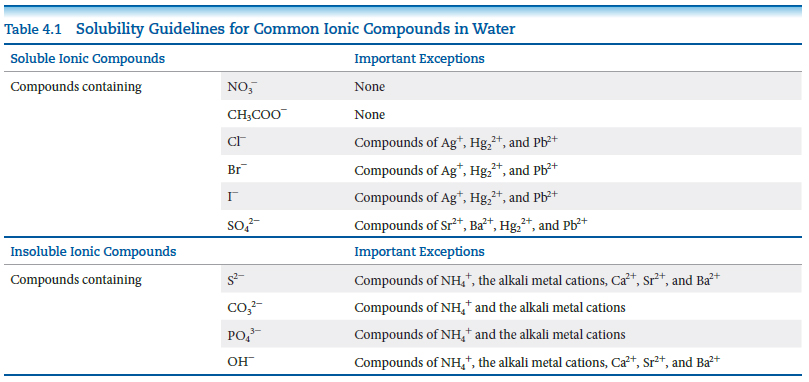# Problem: You choose to investigate some of the solubility guidelines for two ions not listed in the table below, the chromate ion (CrO42 -  and the oxalate ion (C2O42-).You are given 0.01 M solutions (A, B, C, D) of four water-soluble salts:SolutionSoluteColor of SolutionANa2CrO4YellowB(NH4)2C2O4ColorlessCAgNO3ColorlessDCaCl2ColorlessWhen these solutions are mixed, the following observations are made:Experiment NumberSolutions MixedResult1A+BNo precipitate, yellow solution2A+CRed precipitate forms3A+DYellow precipitate forms4B+CWhite precipitate forms5B+DWhite precipitate forms6C+DWhite precipitate formsWrite a net ionic equation for the reaction that occurs in each of the experiments. Identify the precipitate formed, if any, in each of the experiments.Write a net ionic equation for the reaction that occurs in the experiment 4.

###### FREE Expert Solution

Molecular Equation: (NH4)2C2O4(aq) + AgNO3(aq) → NH4NO3(aq) + Ag2C2O4(s)

90% (308 ratings)###### Problem Details
You choose to investigate some of the solubility guidelines for two ions not listed in the table below, the chromate ion (CrO42 -  and the oxalate ion (C2O42-).You are given 0.01 M solutions (A, B, C, D) of four water-soluble salts:

 Solution Solute Color of Solution A Na2CrO4 Yellow B (NH4)2C2O4 Colorless C AgNO3 Colorless D CaCl2 Colorless

When these solutions are mixed, the following observations are made:

 Experiment Number Solutions Mixed Result 1 A+B No precipitate, yellow solution 2 A+C Red precipitate forms 3 A+D Yellow precipitate forms 4 B+C White precipitate forms 5 B+D White precipitate forms 6 C+D White precipitate forms

Write a net ionic equation for the reaction that occurs in each of the experiments. Identify the precipitate formed, if any, in each of the experiments.

Write a net ionic equation for the reaction that occurs in the experiment 4.

Frequently Asked Questions

What scientific concept do you need to know in order to solve this problem?

Our tutors have indicated that to solve this problem you will need to apply the Complete Ionic Equations concept. You can view video lessons to learn Complete Ionic Equations. Or if you need more Complete Ionic Equations practice, you can also practice Complete Ionic Equations practice problems.